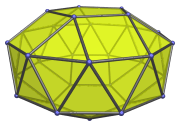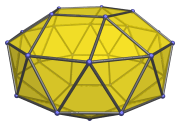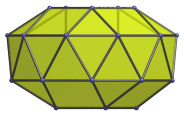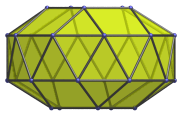# The Gyroelongated Pentagonal Bicupola

The gyroelongated pentagonal bicupola is the 46th Johnson solid (J46). It has 30 vertices, 70 edges, and 42 faces (30 equilateral triangles, 10 squares, and 2 pentagons).The gyroelongated pentagonal bicupola can be constructed by attaching two pentagonal bicupolae to a decagonal antiprism, or equivalently, adding a second pentagonal cupola to a gyroelongated pentagonal cupola (J24).

J46 is one of the few Johnson solids that are chiral; it is distinct from its own mirror image:## Projections

Here are some views of the gyroelongated pentagonal bicupola from various angles:

Projection DescriptionTop view.Front view.9° side view.

## Coordinates

The Cartesian coordinates of the gyroelongated pentagonal bicupola with edge length 2 are:

• (0, √((10+2√(5))/5), 2√((3−φ)/5) + H)
• (±φ, √((5−√(5))/10), 2√((3−φ)/5) + H)
• (±1, −√((5+2√(5))/5), 2√((3−φ)/5) + H)
• (±2φ, 0, H)
• (±1, ±√(3+4φ), H)
• (±φ2, ±√(2+φ), H)
• (0, ±2φ, −H)
• (±√(3+4φ), ±1, −H)
• (±√(2+φ), ±φ2, −H)
• (√((10+2√(5))/5), 0, −(2√((3−φ)/5) + H))
• (√((5−√(5))/10), ±φ, −(2√((3−φ)/5) + H))
• (−√((5+2√(5))/5), ±1, −(2√((3−φ)/5) + H))

where H = √(√(11φ+7)−2φ−1), approximately 0.862397, is half the height of a decagonal antiprism of edge length 2, and φ = (1+√5)/2 is the Golden Ratio.

Last updated 19 Jun 2018.